# SSAT Upper Level Math : How to find the slope of tangent lines

## Example Questions

### Example Question #1 : How To Find The Slope Of A Tangent Line

There is a circle on a coordinate plain. Its perimeter passes through the point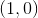. At this point meets a tangent line, which also passes through the point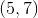. What is the slope of the line perpindicular to this tangent line?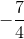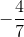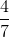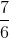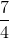Explanation:

In this kind of problem, it's important to keep track of information given about your line of interest. In this case, the coordinates given set up the stage for us to be able to get to our line of focus - the line perpendicular to the tangent line. In order to determine the perpendicular line's slope, the tangent line's slope must be calculated. Keeping in mind that: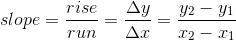where y2,x2 and y1,x1 are assigned arbitrarily as long as the order of assignment is maintained.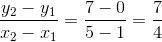which is the slope of the tangent line.

To calculate the perpendicular line, we have to remember that the product of the tangent slope and the perpendicular slope will equal -1.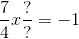, the perpendicular slope can then be calculated as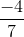### Example Question #1 : How To Find The Slope Of Tangent Lines

Find the slope of a tangent line at point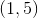if the equation is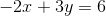.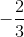Explanation:

Rewrite the linear equation in standard form to slope-intercept form,.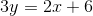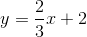Since the slope of every point of this line is, the slope of the tangent line at the given point should also be.